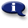# .

## Syllabus Information

Fall 2022
Jun 05,2023Use this page to maintain syllabus information, learning objectives, required materials, and technical requirements for the course.

Syllabus Information
MTH 020 - Math Renewal
Associated Term: Fall 2022
Learning Objectives: Upon successful completion of this course, the student will be able to:
1. Apply reasoning and problem solving skills to basic mathematics problems
2. Use estimation in basic math problems
3. Check the reasonableness of answers
4. Perform fraction computations and applications with accuracy
5. Perform decimal computations and applications with accuracy
4. Write and simplify ratios and rates
6. Recognize when and where ratios or proportions apply
7. Set up and solve proportions
8. Convert between fractions, decimals, and percents
9. Solve the three basic types of percent problems
10. Set up and solve percent application problems
11. Solve applications using area and perimeter of simple geometric shapes
12. Solve basic equations of the type a + x = b and ax = b, involving whole numbers, fractions and decimals
13. Use a scientific calculator to explore and solve basic math problems
14. Apply study skills for learning mathematics and for coping with math anxiety
15. Use the symbols and vocabulary of basic mathematics correctly
16. Use American and metric measurement
Required Materials:
Technical Requirements: